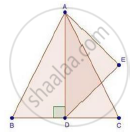# Ad is an Altitude of an Equilateral Triangle Abc. on Ad as Base, Another Equilateral Triangle Ade is Constructed. Prove that Area (δAde): Area (δAbc) = 3: 4 - Mathematics

AD is an altitude of an equilateral triangle ABC. On AD as base, another equilateral triangle ADE is constructed. Prove that Area (ΔADE): Area (ΔABC) = 3: 4

#### SolutionWe have,

ΔABC is an equilateral triangle

Then, AB = BC = AC

Let, AB = BC = AC = 2x

Since, AD ⊥ BC then BD = DC = x

𝐴𝐵2 = (2𝑥)2 − (𝑥)2

⇒ 𝐴𝐷2 = 4𝑥2 − 𝑥2 = 3𝑥2

⇒ 𝐴𝐷 = sqrt3𝑥 cm

Since, ΔABC and ΔADE both are equilateral triangles then they are equiangular

∴ ΔABC ~ ΔADE [By AA similarity]

By area of similar triangle theorem

("area"(triangleADE))/("area"(triangleABC))="AD"^2/"AB"^2

=(sqrt3x)^2/(2x)^2

=(3x^2)/(4x^2)

=3/4

Concept: Areas of Similar Triangles
Is there an error in this question or solution?

#### APPEARS IN

RD Sharma Class 10 Maths
Chapter 7 Triangles
Exercise 7.6 | Q 22 | Page 96